wplogo2

# WORDPRESS里如何输入特殊符号

## 1、输入音标

#### 1.1 安装TypeIt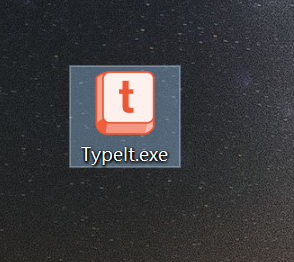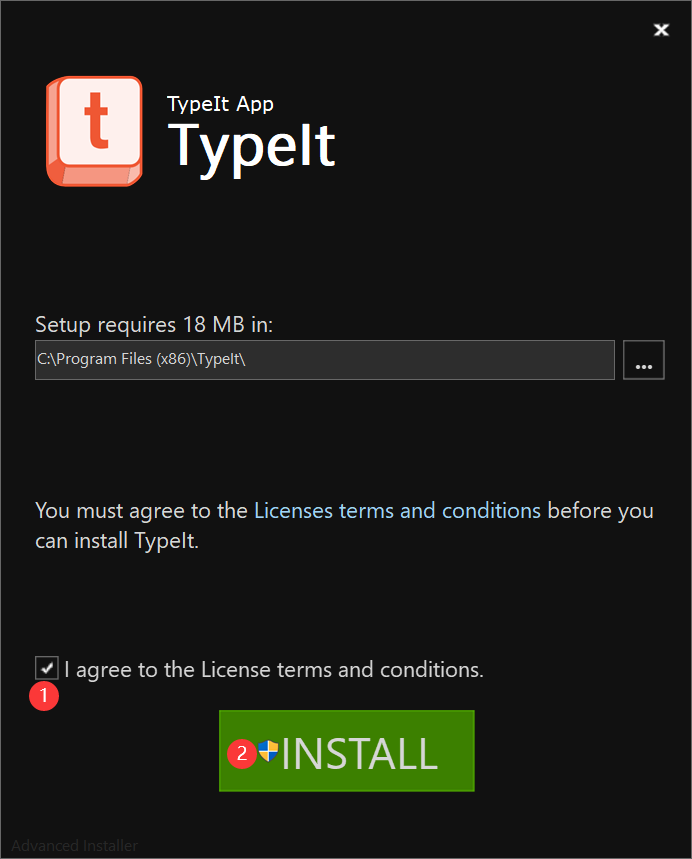#### 1.2 使用TypeIt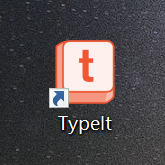## 2、输入数学公式

#### 2.1 插件输入法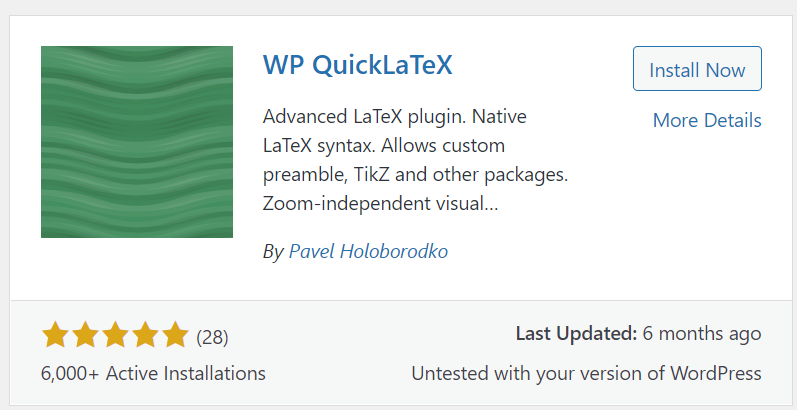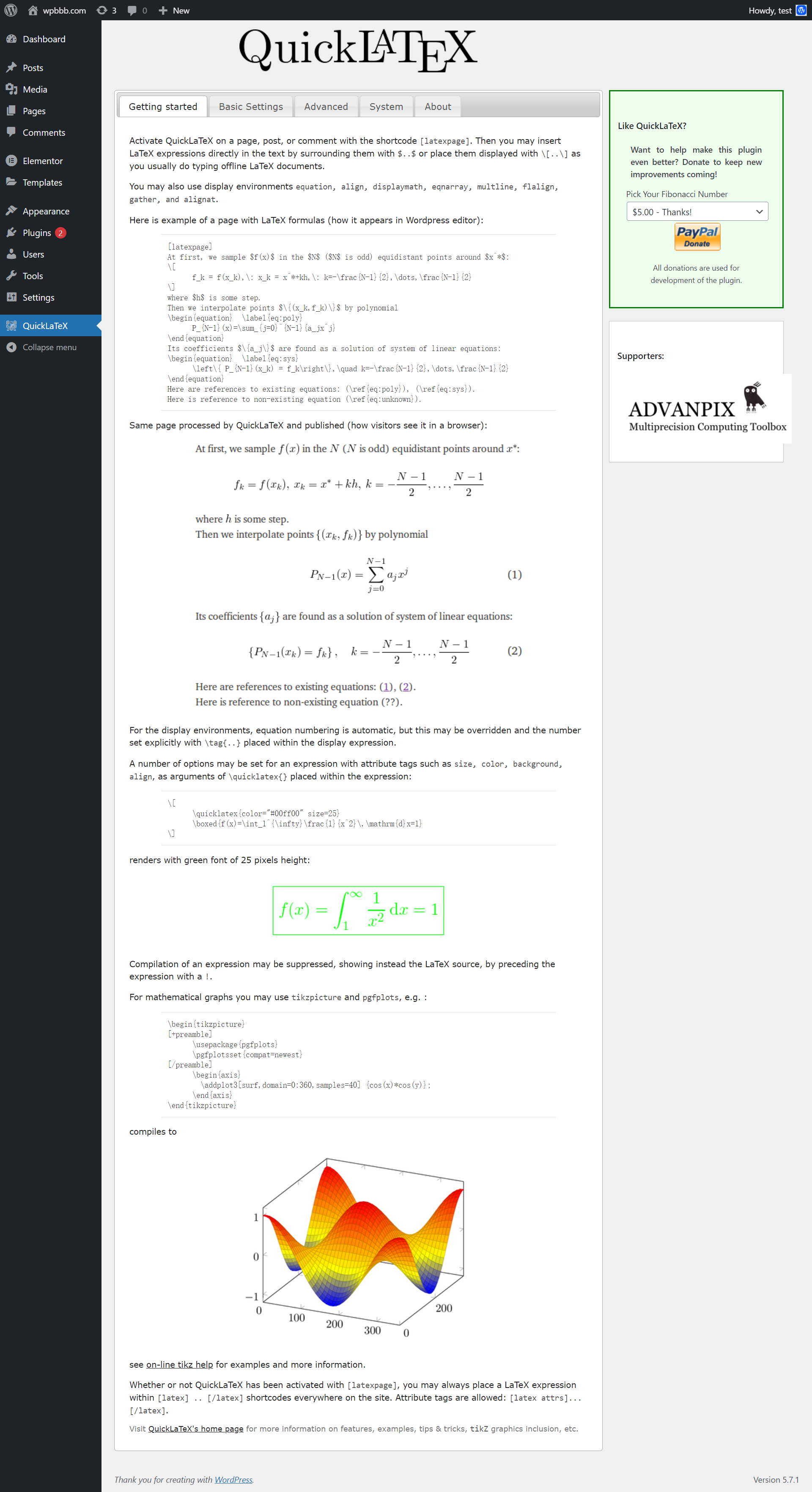x_{1},x_{2}=\frac{-b\pm \sqrt{b^{2}-4ac}}{2a}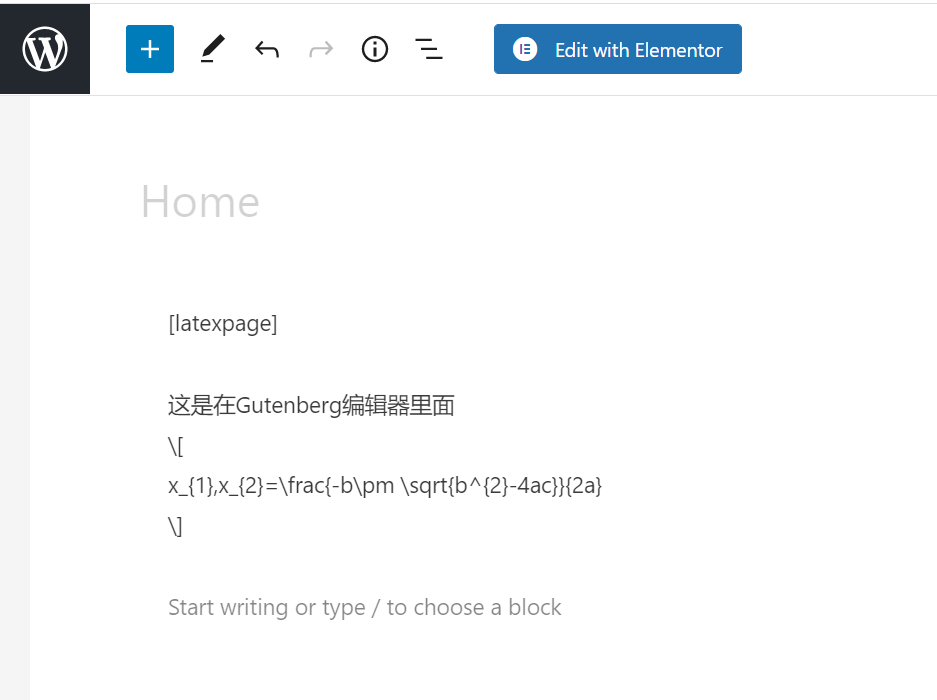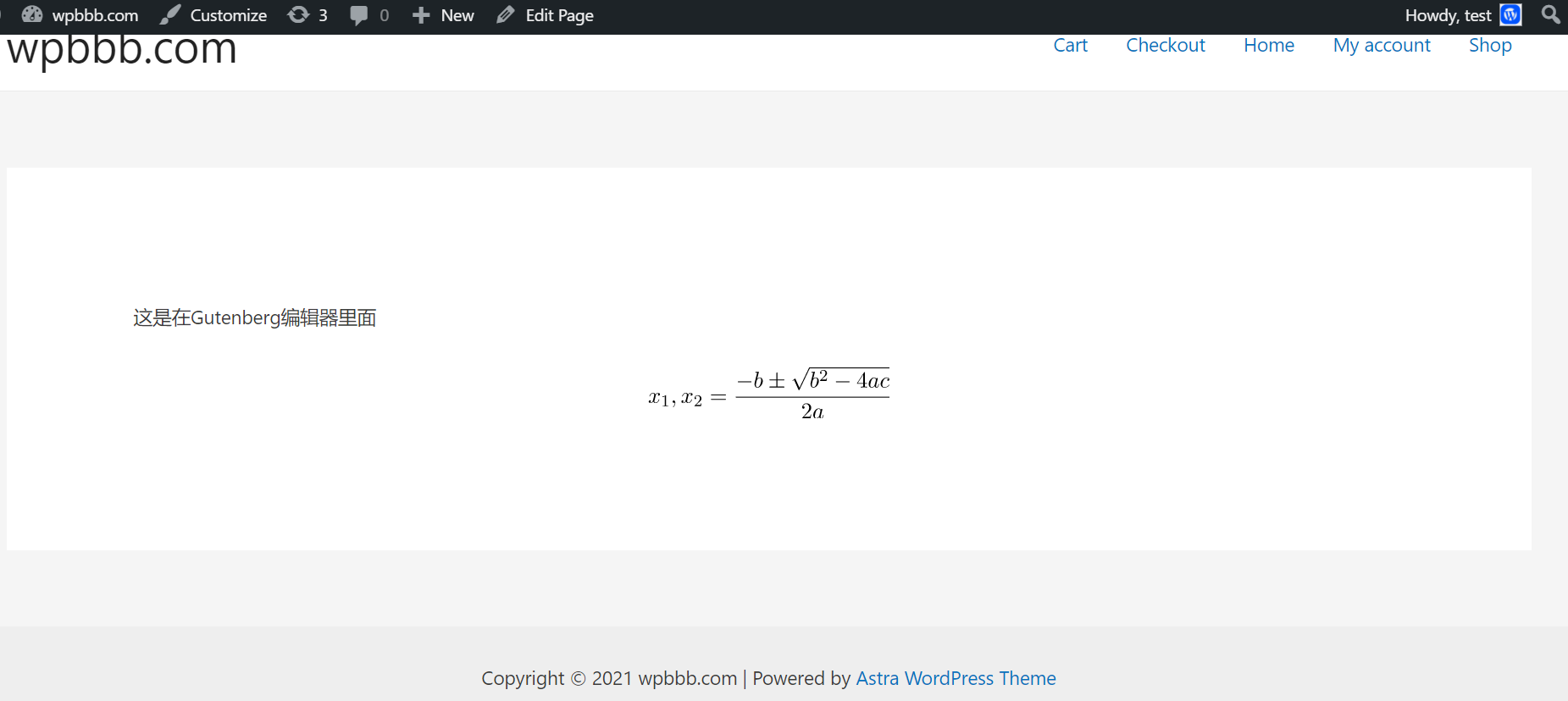[latexpage]

$x_{1},x_{2}=\frac{-b\pm \sqrt{b^{2}-4ac}}{2a}$

$x_{1},x_{2}=\frac{-b\pm \sqrt{b^{2}-4ac}}{2a}$#### 2.2 图片插入法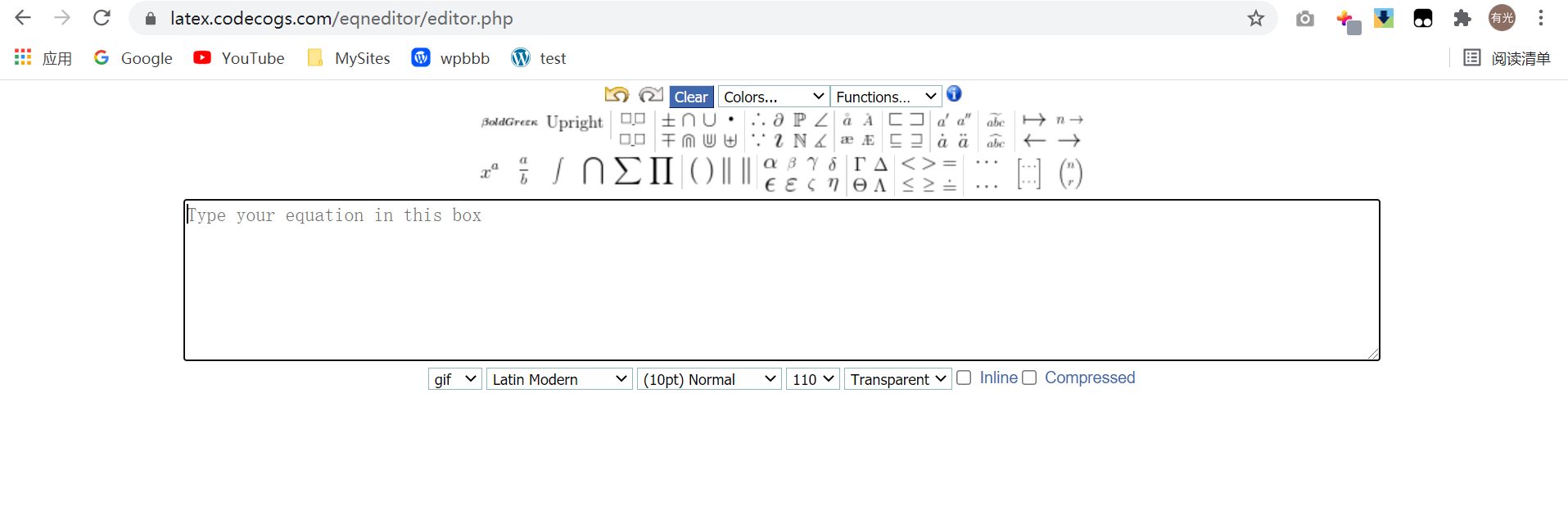x_{1},x_{2}=\frac{-b\pm \sqrt{b^{2}-4ac}}{2a}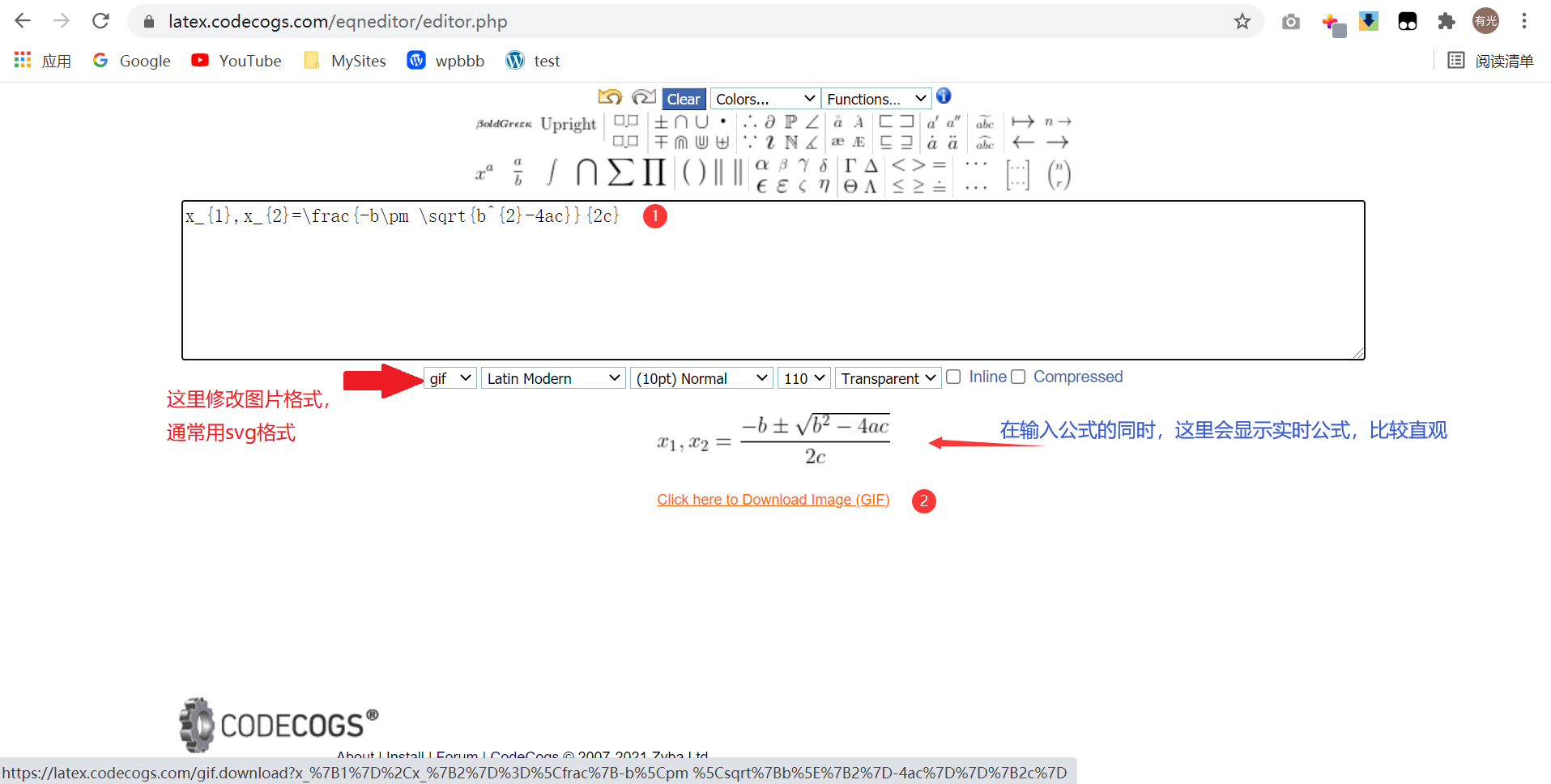#### 2.3 直接输入法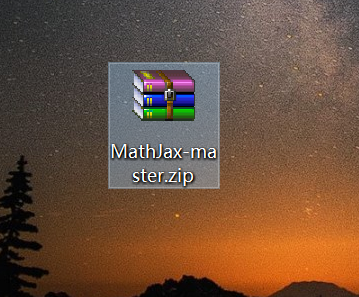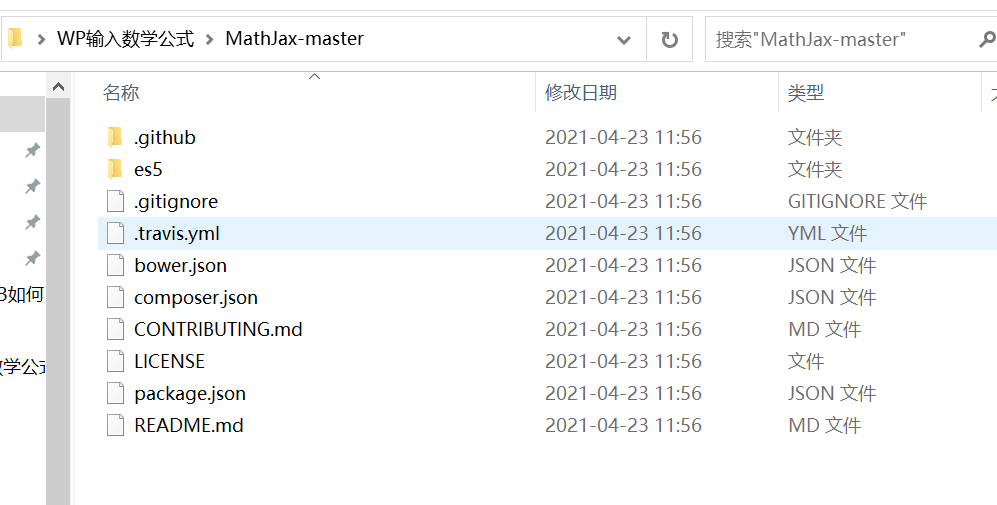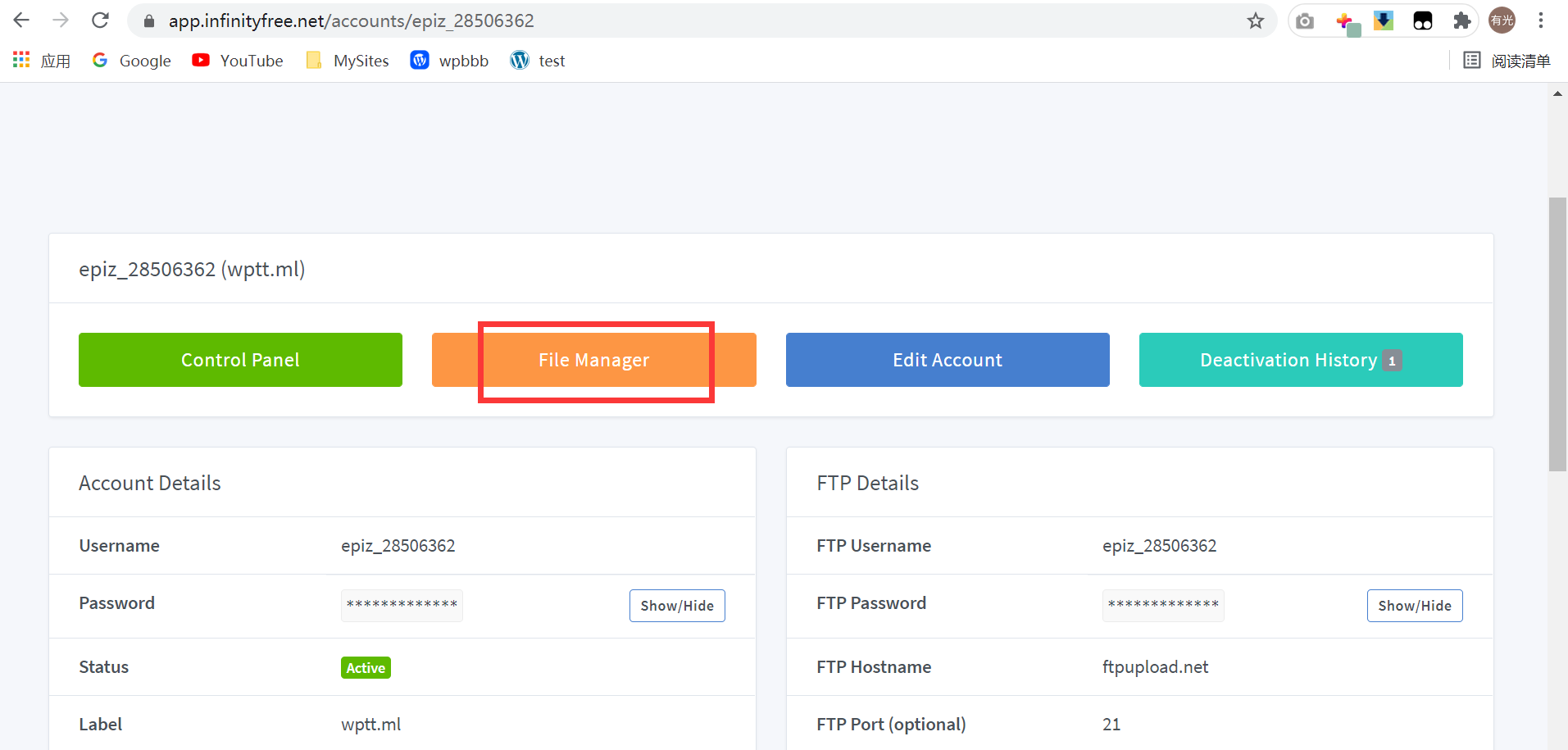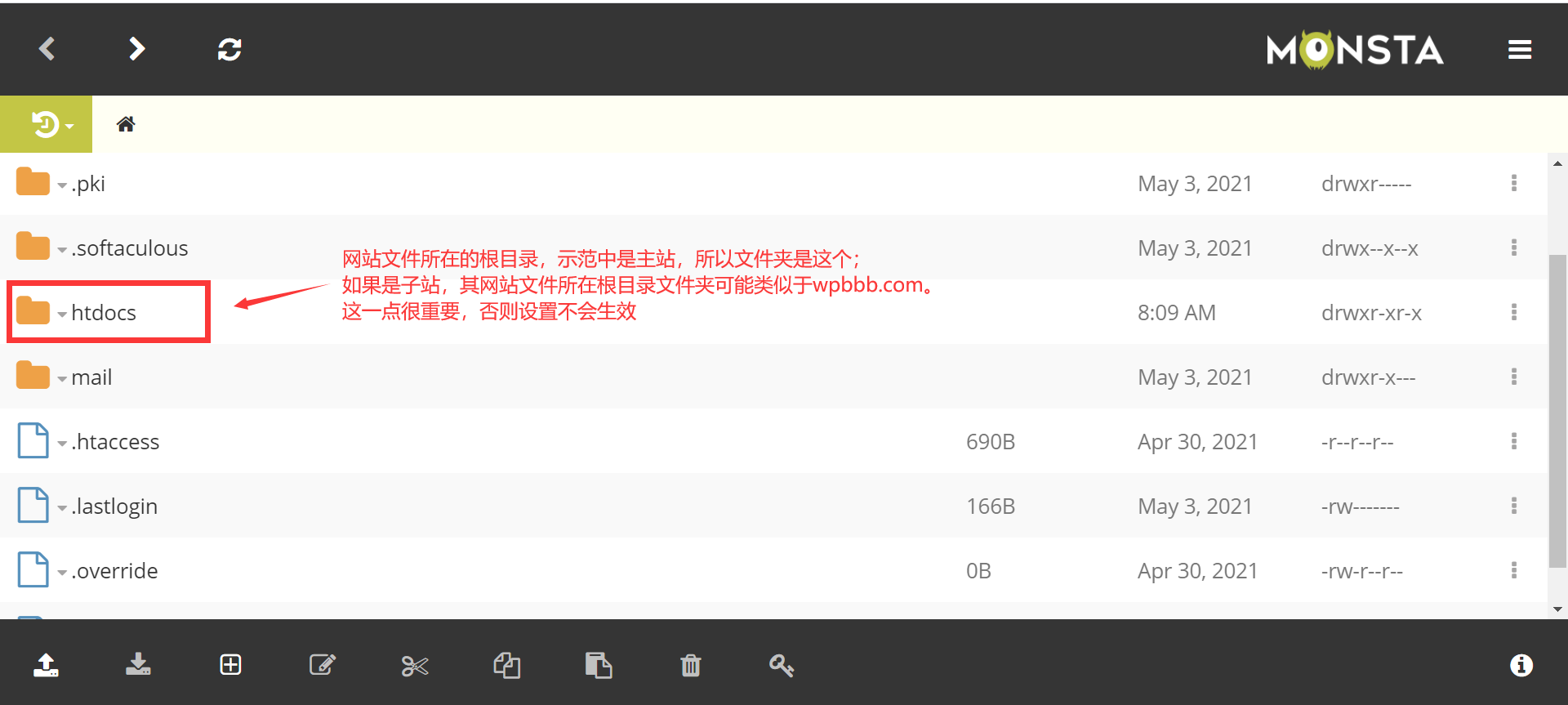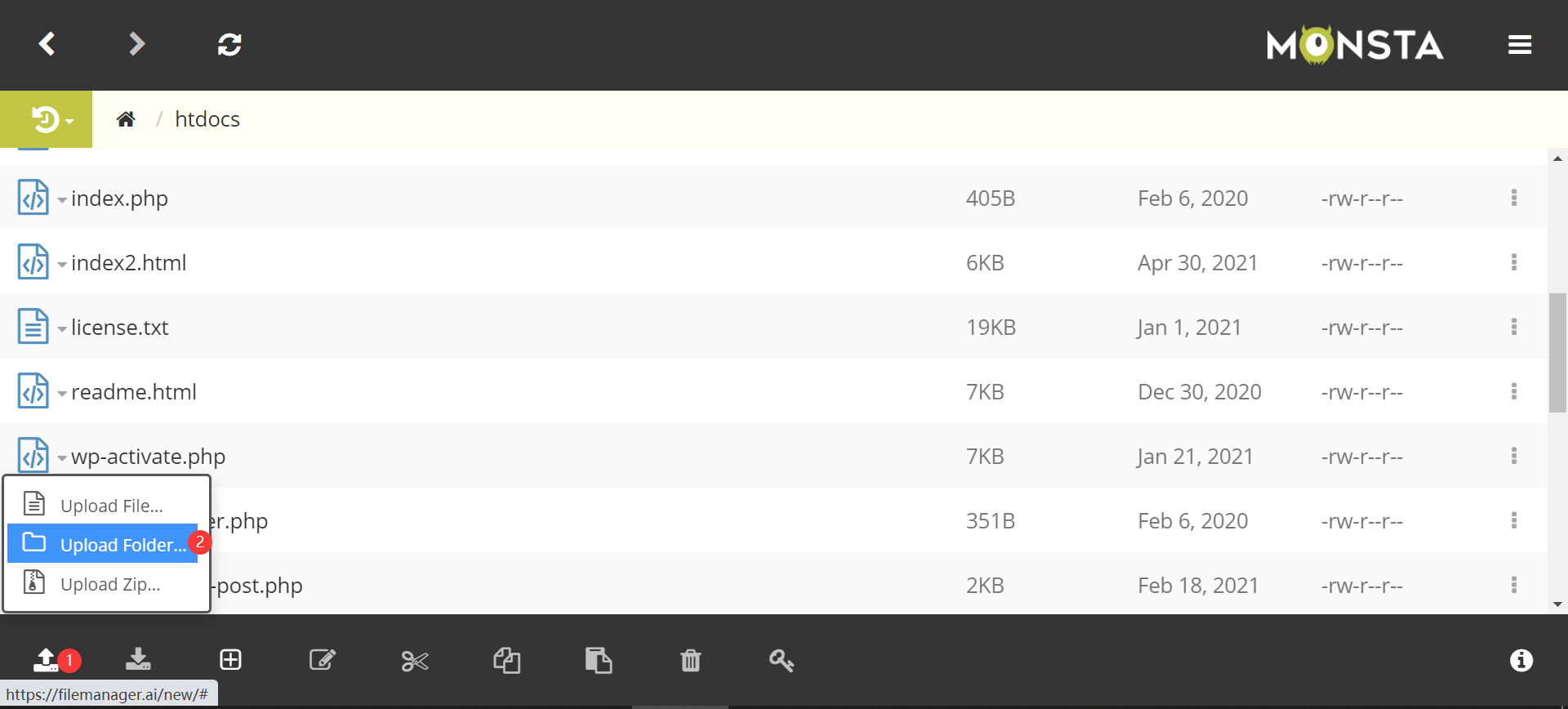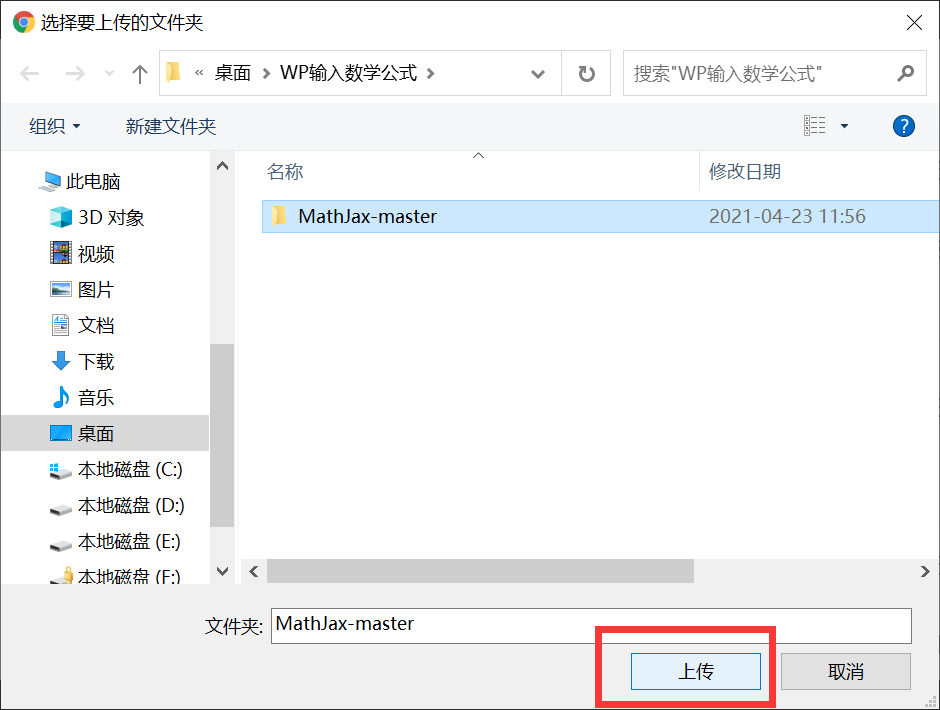99个文件上传完成后，我们可以看到上传好了的文件夹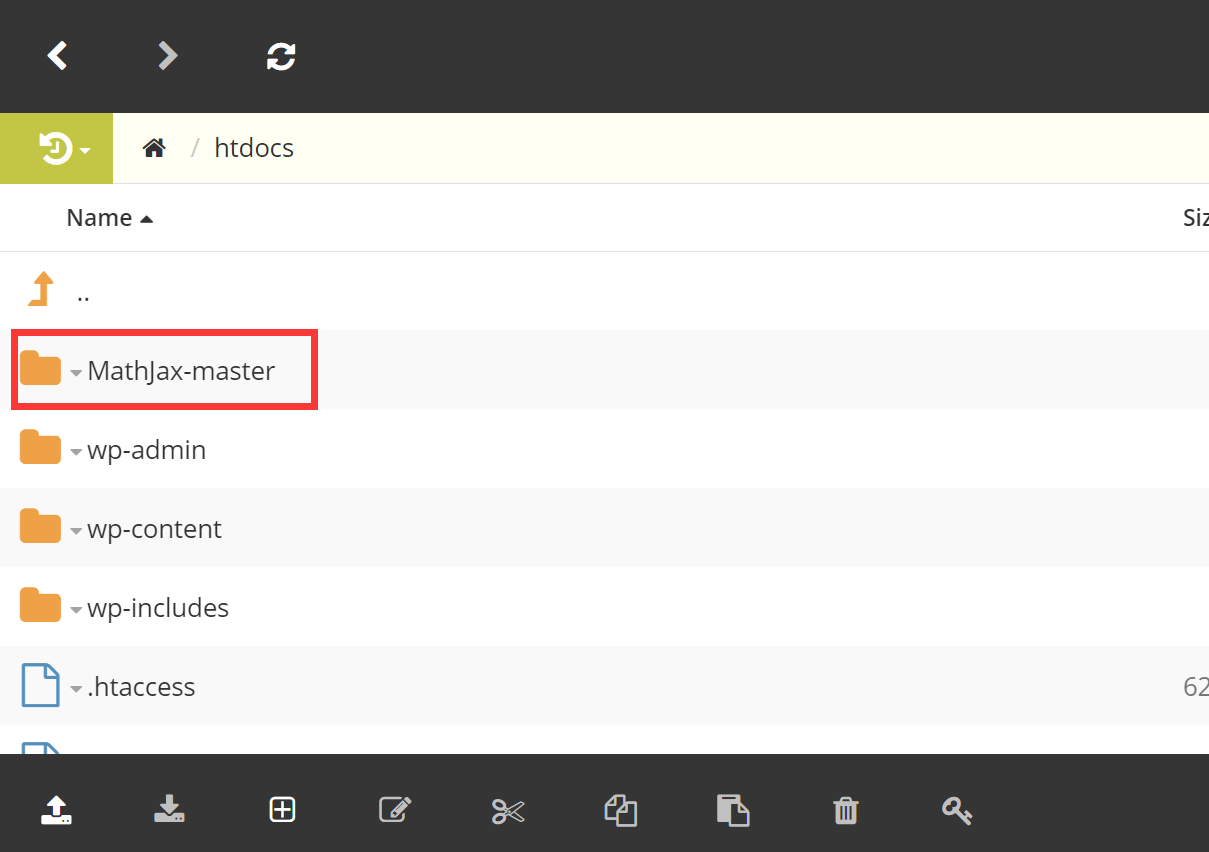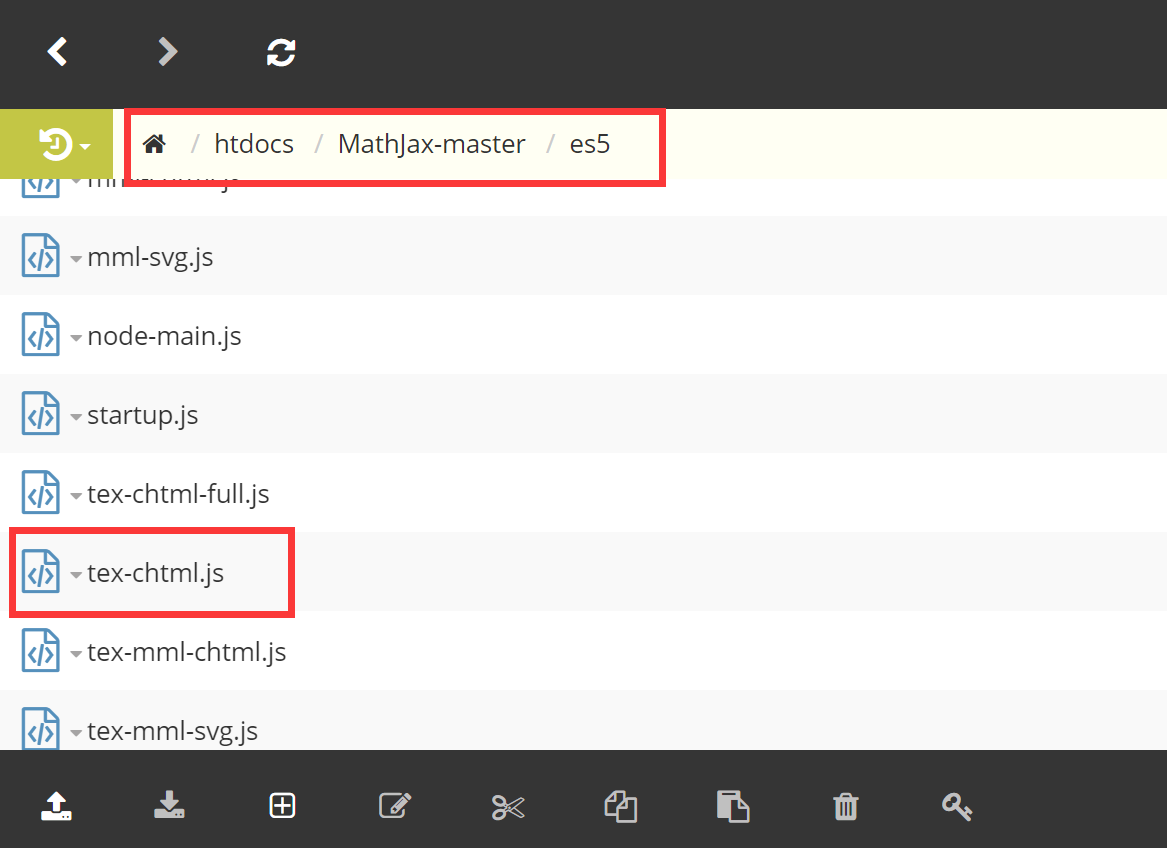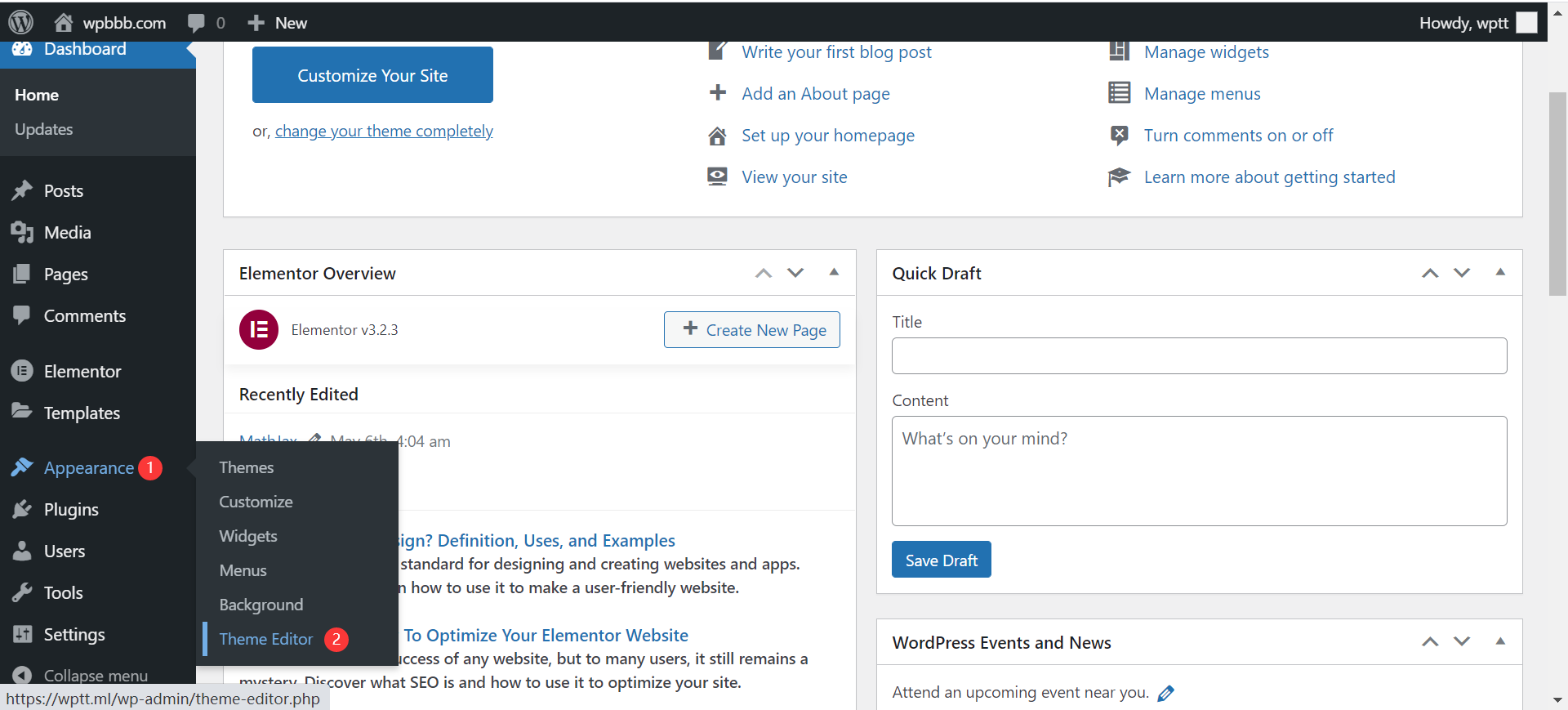src=”/MathJax-master/MathJax-master/es5/tex-chtml.js”